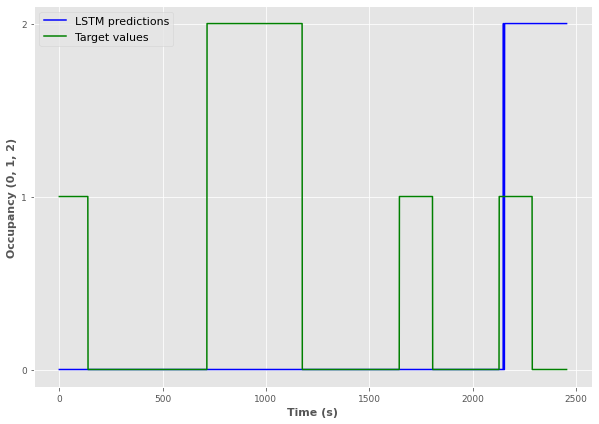# LSTM sequence to label

I’m trying to do occupancy detection with LSTM based on temperature and humidity data as the image shows.

My problem is that I’m getting around 50% accuracy on both of my training and validation dataset under the training. I have tried with different hyperparameters and normalized the dataset etc but I think that the problem is that I don’t really know if my model is implemented correctly. One batch is for example [1, 400, 2] (batch, timesteps, features).

``````class RNN(nn.Module):
def __init__(self, input_size, hidden_layer_size, num_classes):
super(RNN, self).__init__()
self.hidden_layer_size = hidden_layer_size
self.lstm = nn.LSTM(input_size, hidden_layer_size, batch_first=True)
self.fc = nn.Linear(hidden_layer_size, num_classes)

def forward(self, input_seq):
h0 = torch.zeros(1, input_seq.size(0), self.hidden_layer_size).to(device)
c0 = torch.zeros(1, input_seq.size(0), self.hidden_layer_size).to(device)

out, (h_out, _) = self.lstm(input_seq, (h0,c0))
h_out = h_out.view(-1, self.hidden_layer_size)
out = self.fc(h_out)

return out
``````

Do I understand correctly that your target is three classes, i.e., occupancy levels: 0, 1, and 2? Because this would have been my initial suggestion after reading the first sentence.

In general, though, this seems to be a very tricky task. Temperature and humidity are physical phenomena that generally change rather slowly over time – compared to a light or noise sensor, for example. With, say, a noise sensor, you probably wouldn’t even need a RNN.

Sorry, for not really helping here, but I would be curious if you could solve this. At the moment, I see principle limitations with this approach.

Yes, I‘m using 3 target classes (number of occupants 0, 1 or 2) and later under the training I’m using CrossEntropyLoss.
I’m aware of the limitations that you have mentioned but I want to test if it’s a suitable idea for an IoT device.

The image shows the model predictions for the test dataset@Linkan Can you overlay the last plot this with the sensor values?

If I understand correctly, a single data sample is a sequence 400 real values as input and 1 target variable (0, 1, or 2), right?

This is 15 epochs and the accuracy is around 60% (doesn’t look like that from the image).Yes, correct.

I found this function that I used to create the look back sequence for each sample.

``````def TimeSteps(X_data, Y_data, seq_length):
x = []
y = []

for i in range(len(X_data)-seq_length-1):
_x = X_data[i:(i+seq_length)]
_y = Y_data[i+seq_length]
x.append(_x)
y.append(_y)

return np.array(x),np.array(y)
``````